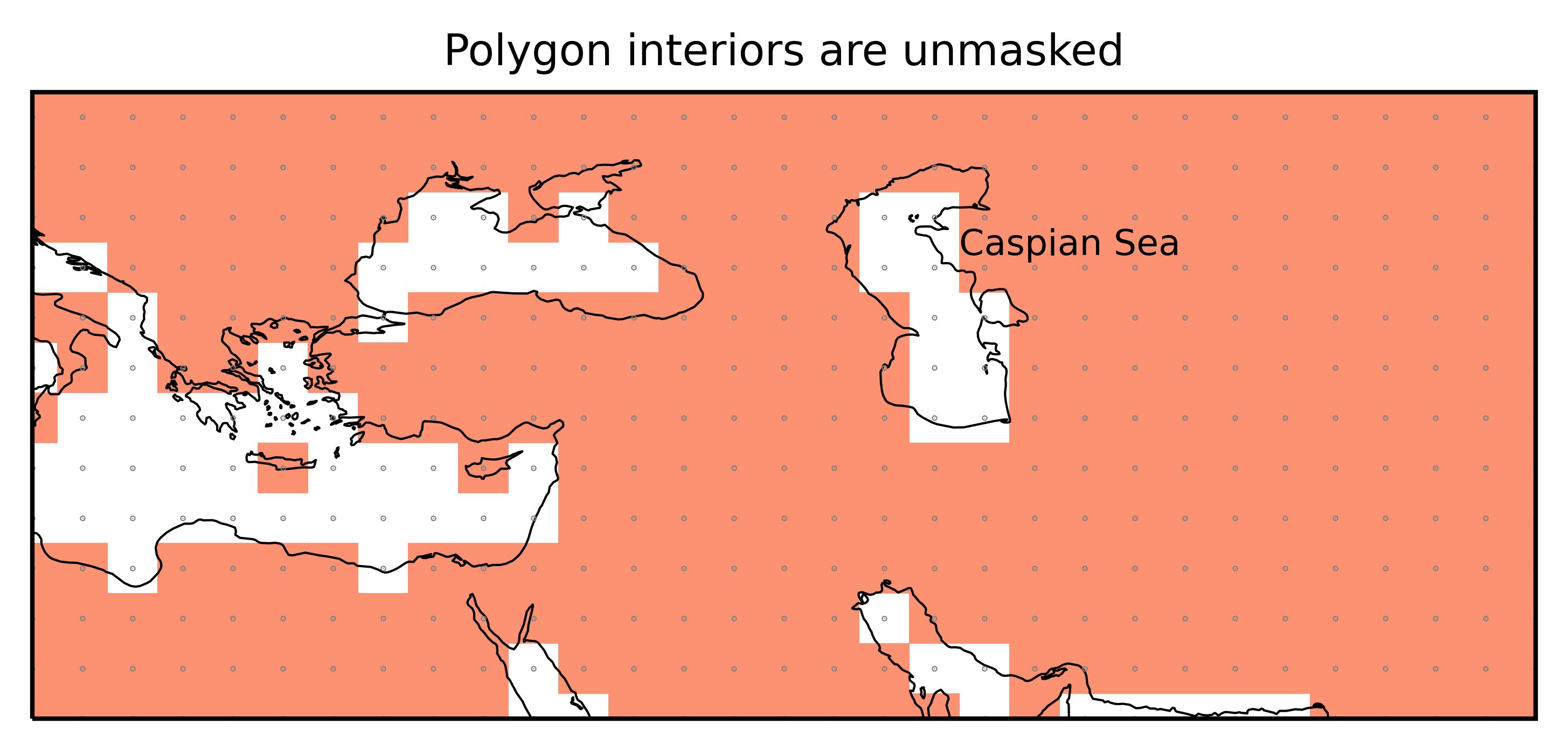None

Note

This tutorial was generated from an IPython notebook that can be downloaded here.

```Matplotlib is building the font cache using fc-list. This may take a moment.
```

# Edge behavior and interiors¶

This notebook illustrates the edge behavior and how Polygon interiors are treated.

Note

From version 0.5 `regionmask` treats points on the region borders differently and also considers poygon interiors (holes), e.g. the Caspian Sea in `natural_earth.land_110` region.

## Preparation¶

Import regionmask and check the version:

```import regionmask

```
```'0.7.0'
```

Other imports

```import xarray as xr
import numpy as np

import cartopy.crs as ccrs
import matplotlib.pyplot as plt

from matplotlib import colors as mplc
from shapely.geometry import Polygon
```

Define some colors:

```cmap1 = mplc.ListedColormap(["#9ecae1"])
cmap2 = mplc.ListedColormap(["#fc9272"])
cmap3 = mplc.ListedColormap(["#cab2d6"])

cmap_2col = mplc.ListedColormap(["#9ecae1", "#fc9272"])
```

## Methods¶

Regionmask offers two methods to rasterize regions

1. `rasterize`: fastest but only for equally-spaced grid, uses `rasterio.features.rasterize` internally.

2. `shapely`: for irregular grids, uses `shapely.vectorized.contains` internally.

All methods use the `lon` and `lat` coordinates to determine if a grid cell is in a region. `lon` and `lat` are assumed to indicate the center of the grid cell. Methods (1) and (2) have the same edge behavior and consider ‘holes’ in the regions. `regionmask` automatically determines which `method` to use.

1. subtracts a tiny offset from `lon` and `lat` to achieve a edge behaviour consistent with (1). Due to mapbox/rasterio/#1844 this is unfortunately also necessary for (1).

## Edge behavior¶

As of version 0.5 `regionmask` has a new edge behavior - points that fall of the outline of a region are now consistently treated. This was not the case in earlier versions (xref matplotlib/matplotlib#9704). It’s easiest to see the edge behaviour in an

### Example¶

Define a region and a lon/ lat grid, such that some gridpoints lie exactly on the border:

```outline = np.array([[-80.0, 50.0], [-80.0, 28.0], [-100.0, 28.0], [-100.0, 50.0]])

```
```ds_US = regionmask.core.utils.create_lon_lat_dataarray_from_bounds(
*(-161, -29, 2), *(75, 13, -2)
)

print(ds_US)
```
```<xarray.Dataset>
Dimensions:   (lon: 65, lat: 30, lon_bnds: 66, lat_bnds: 31)
Coordinates:
* lon       (lon) float64 -160.0 -158.0 -156.0 -154.0 ... -36.0 -34.0 -32.0
* lat       (lat) float64 74.0 72.0 70.0 68.0 66.0 ... 22.0 20.0 18.0 16.0
* lon_bnds  (lon_bnds) int64 -161 -159 -157 -155 -153 ... -39 -37 -35 -33 -31
* lat_bnds  (lat_bnds) int64 75 73 71 69 67 65 63 61 ... 27 25 23 21 19 17 15
LON       (lat, lon) float64 -160.0 -158.0 -156.0 ... -36.0 -34.0 -32.0
LAT       (lat, lon) float64 74.0 74.0 74.0 74.0 ... 16.0 16.0 16.0 16.0
Data variables:
empty```

Let’s create a mask with each of these methods:

```mask_rasterize = region.mask(ds_US, method="rasterize")
```

Note

`regionmask` automatically detects which method to use, so there is no need to specify the `method` keyword.

```f, axes = plt.subplots(1, 2, subplot_kw=dict(projection=ccrs.PlateCarree()))

opt = dict(add_colorbar=False, ec="0.5", lw=0.5, transform=ccrs.PlateCarree())

for ax in axes:
ax.set_extent([-105, -75, 25, 55], ccrs.PlateCarree())
ax.coastlines(lw=0.5)

ax.plot(
ds_US.LON, ds_US.lat, "*", color="0.5", ms=0.5, transform=ccrs.PlateCarree()
)

axes.set_title("rasterize")
axes.set_title("shapely")
```
```Text(0.5, 1.0, 'shapely')
```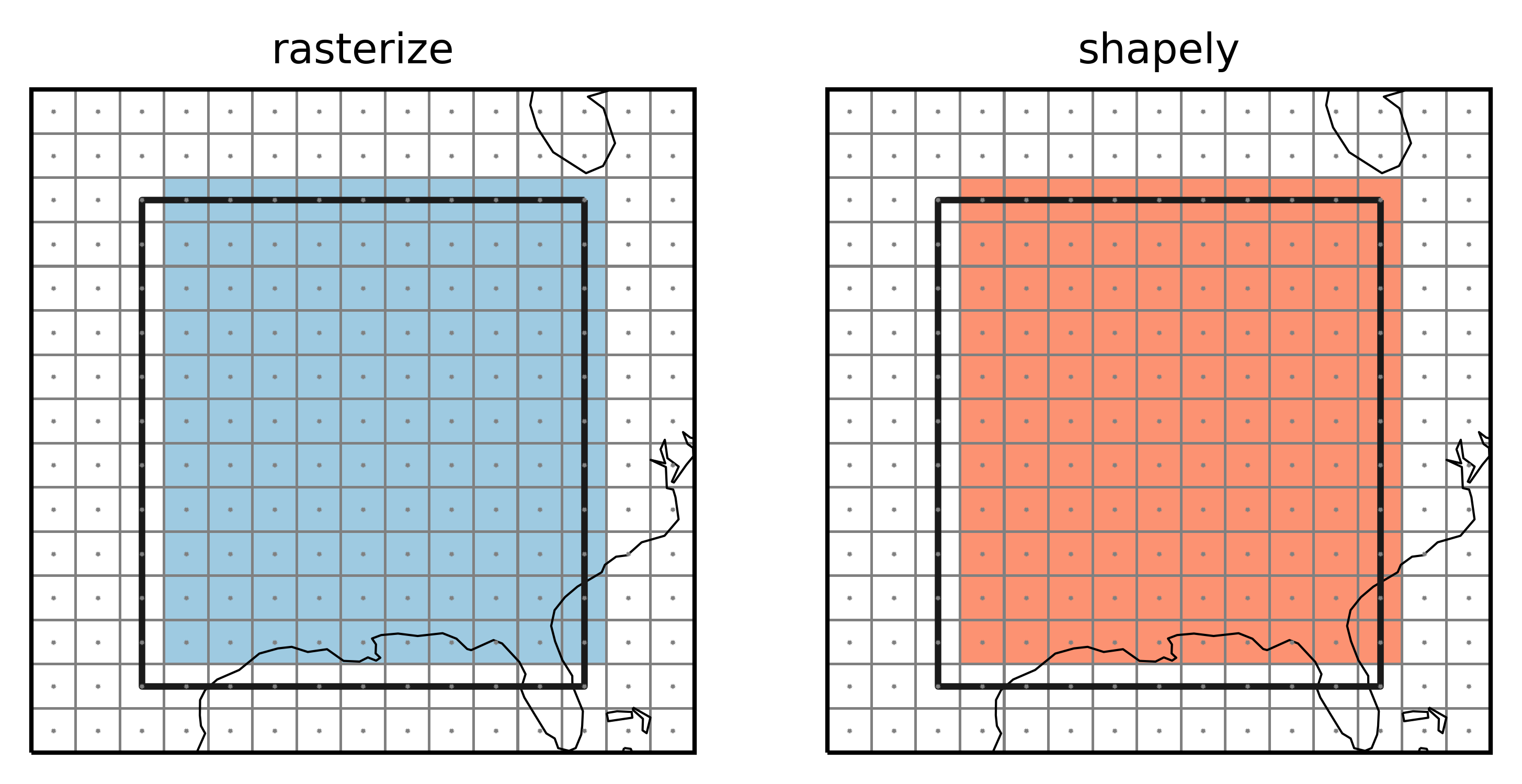Points indicate the grid cell centers (`lon` and `lat`), lines the grid cell borders, colored grid cells are selected to be part of the region. The top and right grid cells now belong to the region while the left and bottom grid cells do not. This choice is arbitrary but mimicks what `rasterio.features.rasterize` does. This avoids spurious columns of unassigned grid points as the following example shows.

### SREX regions¶

Create a global dataset:

```ds_GLOB = regionmask.core.utils.create_lon_lat_dataarray_from_bounds(
*(-180, 181, 2), *(90, -91, -2)
)
```
```srex = regionmask.defined_regions.srex

```
```f, ax = plt.subplots(1, 1, subplot_kw=dict(projection=ccrs.PlateCarree()))

srex_new.plot(ax=ax, ec="0.7", lw=0.25, **opt)

ax.set_extent([-135, -50, 24, 51], ccrs.PlateCarree())
ax.coastlines(resolution="50m", lw=0.25)

ax.plot(
ds_GLOB.LON, ds_GLOB.lat, "*", color="0.5", ms=0.5, transform=ccrs.PlateCarree()
)

sel = ((ds_GLOB.LON == -105) | (ds_GLOB.LON == -85)) & (ds_GLOB.LAT > 28)

ax.plot(
ds_GLOB.LON.values[sel],
ds_GLOB.LAT.values[sel],
"*",
color="r",
ms=0.5,
transform=ccrs.PlateCarree(),
)

ax.set_title("new (rasterize + shapely)");
```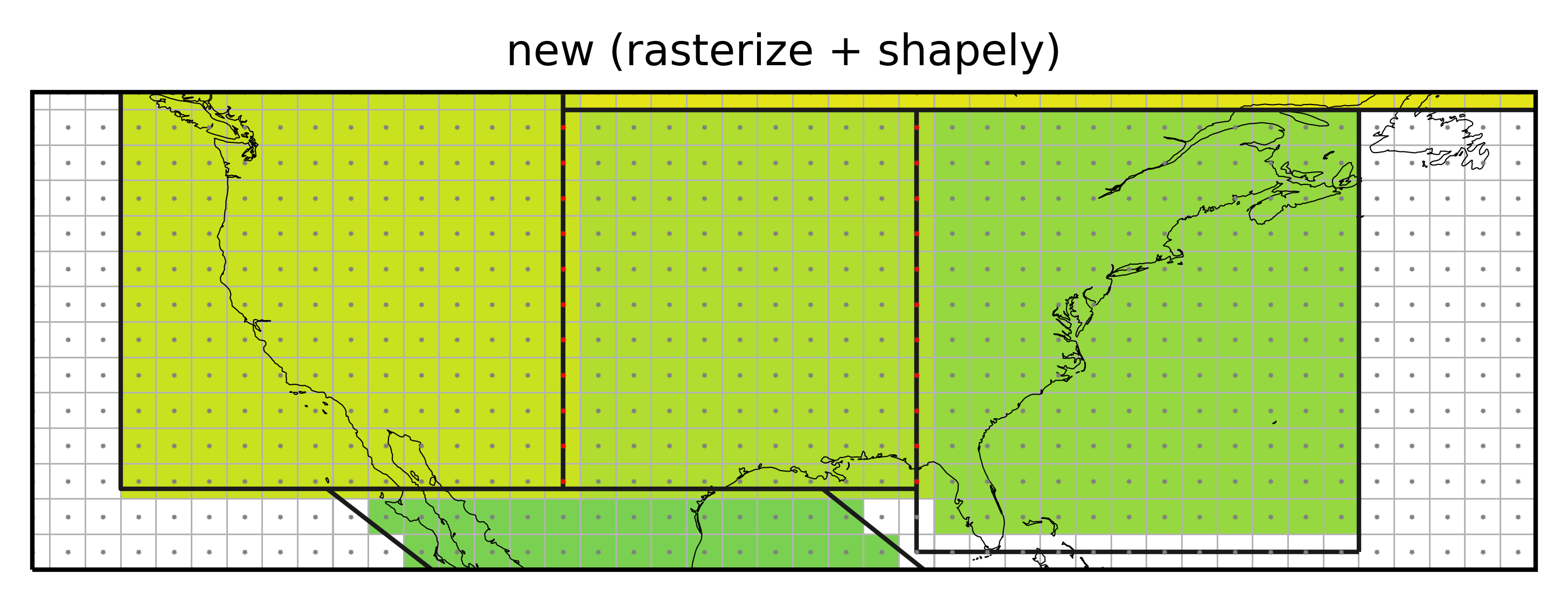Not assigning the grid cells falling exactly on the border of a region (red points) would leave vertical stripes of unassigned cells.

### Points at -180°E (0°E) and -90°N¶

The described edge behaviour leads to a consistent treatment of points on the border. However, gridpoints at -180°E (or 0°E) and -90°N would never fall in any region.

We exemplify this with a region spanning the whole globe and a coarse longitude/ latidude grid:

```outline_global = np.array(
[[-180.0, 90.0], [-180.0, -90.0], [180.0, -90.0], [180.0, 90.0]]
)

lon = np.arange(-180, 180, 30)
lat = np.arange(90, -91, -30)

LON, LAT = np.meshgrid(lon, lat)
```

```mask_global = region_global.mask(lon, lat)

# we need to manually create the mask
```

And illustrate the issue:

```f, axes = plt.subplots(1, 2, subplot_kw=dict(projection=ccrs.PlateCarree()))

opt = dict(add_colorbar=False, ec="0.3", lw=0.5, transform=ccrs.PlateCarree())

ax = axes

# only for the gridlines

ax.set_title("Not treating points at -180°E and -90°N", size=6)
ax.set_title("(a)", loc="left", size=6)

ax = axes

ax.set_title("Treating points at -180°E and -90°N", size=6)
ax.set_title("(b)", loc="left", size=6)

for ax in axes:

ax = region_global.plot(
)
ax.plot(LON, LAT, "o", color="0.3", ms=1, transform=ccrs.PlateCarree(), zorder=5)

ax.outline_patch.set_visible(False)
```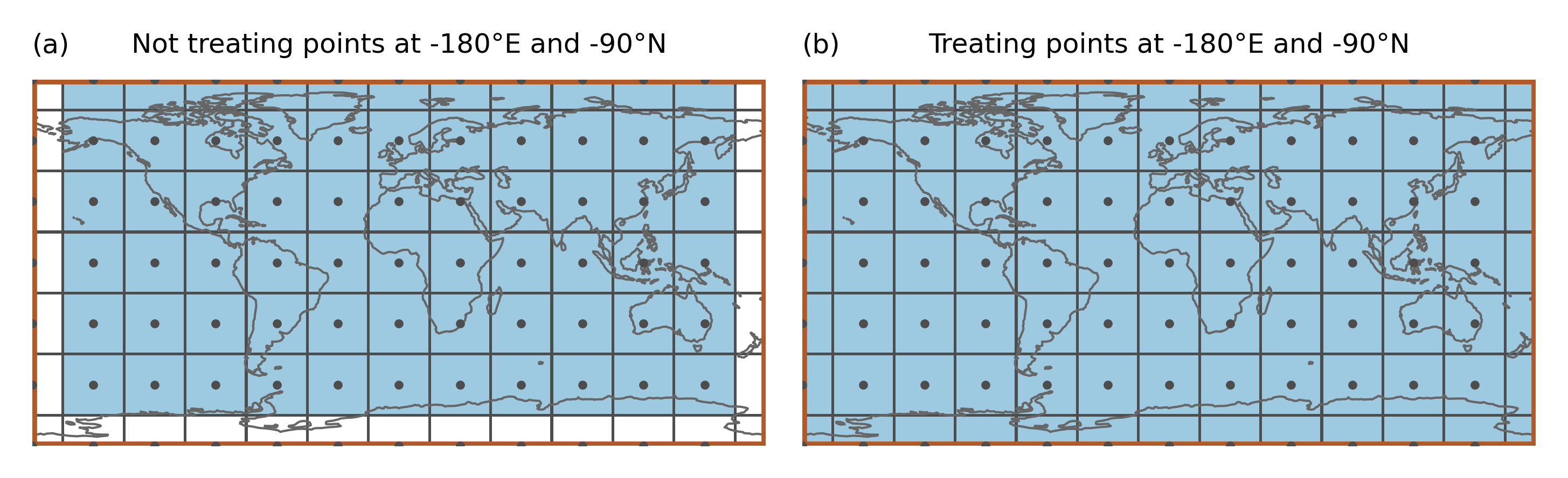In the example the region spans the whole globe and there are gridpoints at -180°E and -90°N. Just applying the approach above leads to gridpoints that are not assigned to any region even though the region is global (as shown in a). Therefore, points at -180°E (or 0°E) and -90°N are treated specially (b):

Points at -180°E (0°E) are mapped to 180°E (360°E). Points at -90°N are slightly shifted northwards (by 1 * 10 ** -10). Then it is tested if the shifted points belong to any region

This means that (i) a point at -180°E is part of the region that is present at 180°E (and not the one at -180°E) and (ii) only the points at -90°N get assigned to the region above.

```outline_global1 = np.array([[-180.0, 60.0], [-180.0, -60.0], [0.0, -60.0], [0.0, 60.0]])
outline_global2 = np.array([[0.0, 60.0], [0.0, -60.0], [180.0, -60.0], [180.0, 60.0]])

```
```ax = region_global_2.plot(line_kws=dict(color="#b15928", zorder=3), add_label=False,)

ax.plot(LON, LAT, "o", color="0.3", ms=2, transform=ccrs.PlateCarree(), zorder=5)

# only for the gridlines

ax.set_title("Points at -180°E are mapped to 180°E", size=6)

ax.outline_patch.set_lw(0.5)
ax.outline_patch.set_zorder(1);
```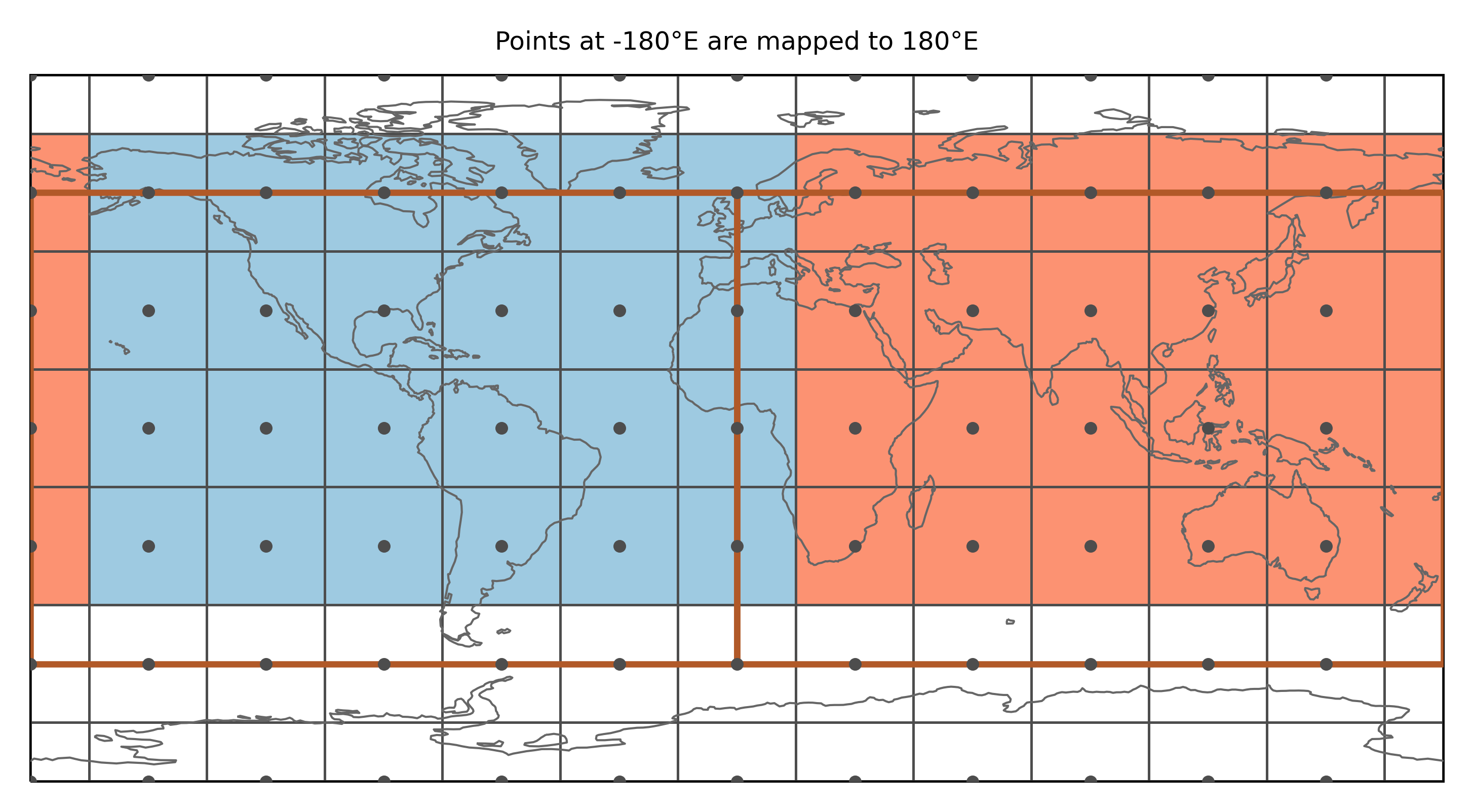Note

This only applies if the border of the region falls exactly on the point. One way to avoid the problem is to calculate the fractional overlap of each gridpoint with the regions (which is not yet implemented).

## Polygon interiors¶

`Polygons` can have interior boundaries (‘holes’). Prior to version 0.5.0 these were not considered and e.g. the Caspian Sea was not ‘unmasked’.

### Example¶

Let’s test this on an example and define a `region_with_hole`:

```interior = np.array(
[[-86.0, 44.0], [-86.0, 34.0], [-94.0, 34.0], [-94.0, 44.0], [-86.0, 44.0],]
)

poly = Polygon(outline, [interior])

```
```mask_hole_rasterize = region_with_hole.mask(ds_US, method="rasterize")
```
```f, axes = plt.subplots(1, 2, subplot_kw=dict(projection=ccrs.PlateCarree()))

for ax in axes:

ax.set_extent([-105, -75, 25, 55], ccrs.PlateCarree())
ax.coastlines(lw=0.5)

ax.plot(
ds_US.LON, ds_US.lat, "o", color="0.5", ms=0.5, transform=ccrs.PlateCarree()
)

axes.set_title("rasterize")
axes.set_title("shapely");
```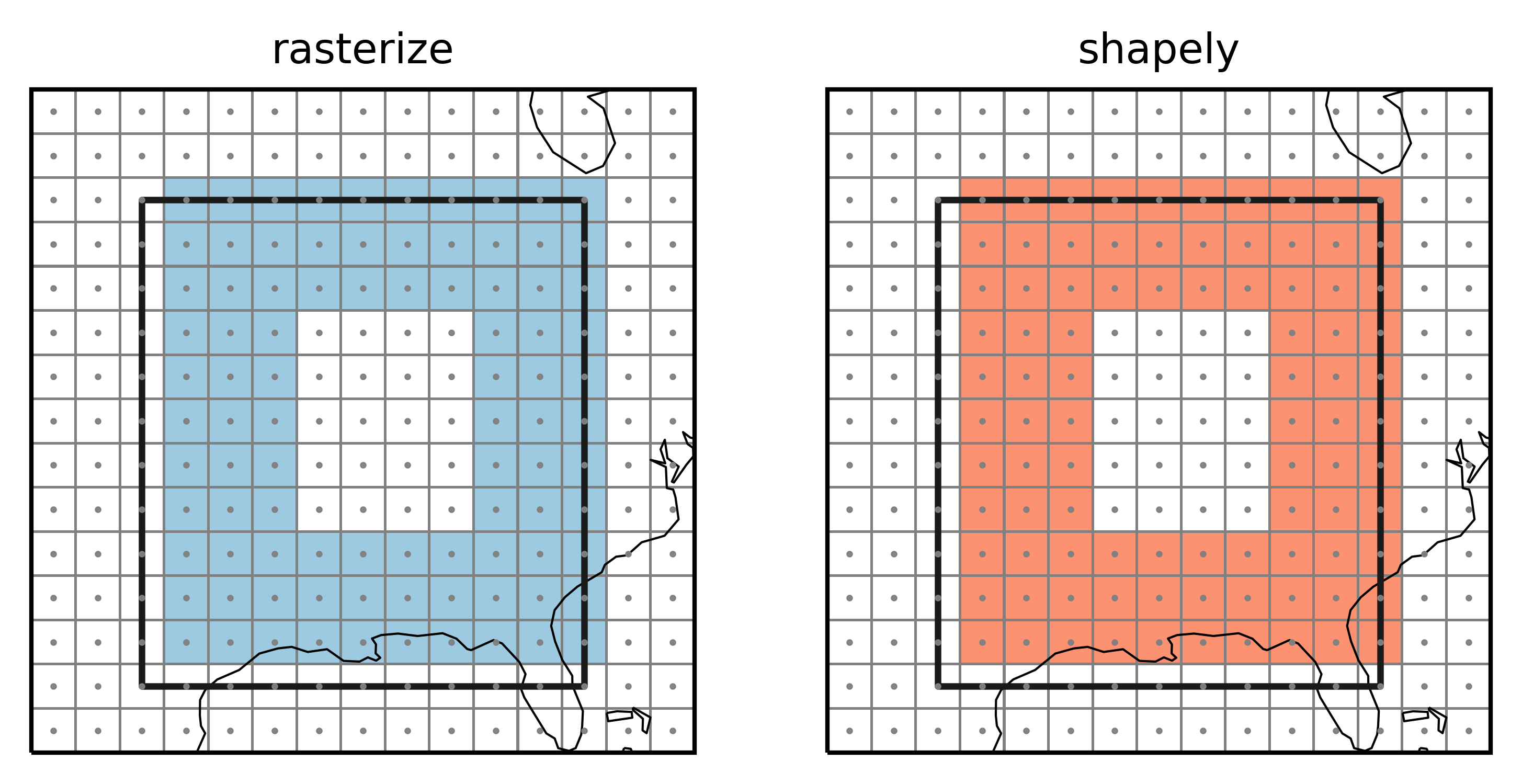### Caspian Sea¶

```land110 = regionmask.defined_regions.natural_earth.land_110

```
```f, ax = plt.subplots(1, 1, subplot_kw=dict(projection=ccrs.PlateCarree()))

land_new.plot(ax=ax, cmap=cmap2, **opt)

ax.set_extent([15, 75, 25, 50], ccrs.PlateCarree())
ax.coastlines(resolution="50m", lw=0.5)

ax.plot(
ds_GLOB.LON, ds_GLOB.lat, ".", color="0.5", ms=0.5, transform=ccrs.PlateCarree()
)

ax.text(52, 43.5, "Caspian Sea", transform=ccrs.PlateCarree())